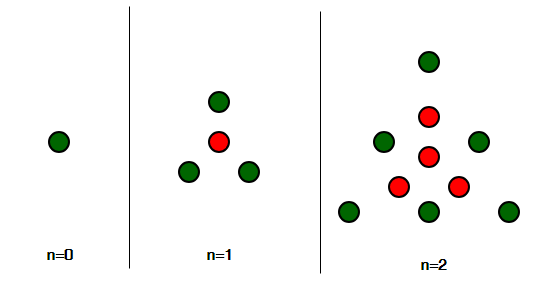# Centered triangular number

Given an integer n, find the nth Centered triangular number.

Centered Triangular Number is a centered polygonal number that represents a triangle with a dot in the centre and all other dots surrounding the centre in successive triangular layers [Source : Wiki ]

Pictorial Representation :The first few centered triangular number series are :
1, 4, 10, 19, 31, 46, 64, 85, 109, 136, 166, 199, 235, 274, 316, 361, 409, 460………………………..

Examples:

```Input : n = 1
Output : 4
Explanation :
A dot in the centre and 3 dots forming the
triangle outside it, thus 4.

Input : n = 6
Output : 64

Input : n = 10
Output : 166
```

## Recommended: Please try your approach on {IDE} first, before moving on to the solution.

Approach
nth Term of centered triangular number is given by:

``````

Basic Implementation of the above approach:

## C++

 `// CPP Program to find the ` `// nth Centered Trigunal number ` `#include  ` `using` `namespace` `std; ` ` `  `// function for Centered ` `// Trigunal number ` `int` `Centered_Trigunal_num(``int` `n) ` `{ ` `    ``// formula for find Centered ` `    ``// Trigunal number nth term ` `    ``return` `(3 * n * n + 3 * n + 2) / 2; ` `} ` ` `  `// Driver Code ` `int` `main() ` `{ ` `    ``// For 3rd Centered Trigunal number ` `    ``int` `n = 3; ` `    ``cout << Centered_Trigunal_num(n) << endl; ` ` `  `    ``// For 12th Centered Trigunal number ` `    ``n = 12; ` `    ``cout << Centered_Trigunal_num(n) << endl; ` ` `  `    ``return` `0; ` `} `

## Java

 `// Java Program to find  ` `// the nth Centered  ` `// Triangular number ` `import` `java.io.*; ` ` `  `class` `GFG  ` `{ ` `     `  `// function for Centered ` `// Trigunal number ` `static` `int` `Centered_Trigunal_num(``int` `n) ` `{ ` `    ``// formula for find Centered ` `    ``// Trigunal number nth term ` `    ``return` `(``3` `* n * n +  ` `            ``3` `* n + ``2``) / ``2``; ` `} ` ` `  `// Driver Code ` `public` `static` `void` `main (String[] args)  ` `{ ` ` `  `// For 3rd Centered  ` `// Trigunal number ` `int` `n = ``3``; ` `System.out.println(Centered_Trigunal_num(n)); ` ` `  `// For 12th Centered  ` `// Trigunal number ` `n = ``12``; ` `System.out.println(Centered_Trigunal_num(n)); ` `} ` `} ` ` `  `// This code is contributed by ajit `

## Python3

 `# Program to find nth  ` `# Centered Trigunal number ` ` `  `def` `Centered_Trigunal_num(n) : ` `     `  `    ``# Formula to calculate nth ` `    ``# Centered Trigunal number ` `    ``return` `(``3` `*` `n ``*` `n ``+`  `            ``3` `*` `n ``+` `2``) ``/``/` `2` ` `  `# Driver Code ` `if` `__name__ ``=``=` `'__main__'` `: ` ` `  `    ``# For 3rd Centered  ` `    ``# Trigunal number      ` `    ``n ``=` `3` `    ``print``(Centered_Trigunal_num(n)) ` `     `  `    ``# For 12th Centered ` `    ``# Trigunal number ` `    ``n ``=` `12` `    ``print``(Centered_Trigunal_num(n)) ` `                 `  `                 `  `# This code is contributed  ` `# by akt_mit `

## C#

 `// C# Program to find  ` `// the nth Centered  ` `// Triangular number ` `using` `System; ` ` `  `class` `GFG ` `{ ` ` `  `// function for Centered ` `// Trigunal number ` `static` `int` `Centered_Trigunal_num(``int` `n) ` `{ ` `    ``// formula for find Centered ` `    ``// Trigunal number nth term ` `    ``return` `(3 * n * n +  ` `            ``3 * n + 2) / 2; ` `} ` ` `  `// Driver Code ` `static` `public` `void` `Main () ` `{ ` ` `  `// For 3rd Centered  ` `// Trigunal number ` `int` `n = 3; ` `Console.WriteLine(Centered_Trigunal_num(n)); ` ` `  `// For 12th Centered  ` `// Trigunal number ` `n = 12; ` `Console.WriteLine(Centered_Trigunal_num(n)); ` `} ` `} ` ` `  `// This code is contributed by akt_mit `

## PHP

 ` `

Output :

```19
235
```

My Personal Notes arrow_drop_upCheck out this Author's contributed articles.

If you like GeeksforGeeks and would like to contribute, you can also write an article using contribute.geeksforgeeks.org or mail your article to contribute@geeksforgeeks.org. See your article appearing on the GeeksforGeeks main page and help other Geeks.

Please Improve this article if you find anything incorrect by clicking on the "Improve Article" button below.

Improved By : jit_t

Article Tags :
Practice Tags :

1

Please write to us at contribute@geeksforgeeks.org to report any issue with the above content.